# Difference Between Moment of Inertia and Polar Moment of Inertia: Notes pdf ppt Engineering Mechanics

## Main Difference – Moment of Inertia vs. Polar Moment of Inertia:

Moment of inertia (which refers to the mass second moment of inertia) and polar (second) moment of inertia are both quantities which describe an object’s ability to resist changes due to torques applied to it. The main difference between moment of inertia and polar moment of inertia is that the moment of inertia measures how an object resists angular acceleration, whereas the polar moment of inertia measures how an object resists torsion.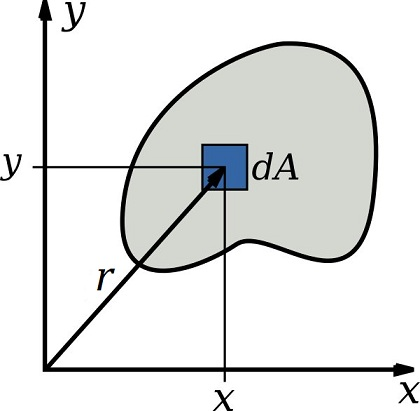## What is Moment of Inertia:

Moment of inertia for a body is defined with the following integral: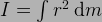where$r$ is the distance of the mass element$\mathrm dm$ from the axis of rotation.

Physically, the moment of inertia gives the ability of a body to resist angular acceleration (analogous to how mass resists linear acceleration) when subject to a torque. If the moment of inertia is large, then the angular acceleration produced by a given torque would be smaller. The unit of moment of inertia is kg m2.

Moment of inertia is used to calculate rotational kinetic energy and also to calculate torque in terms of mass and angular acceleration.

## What is Polar Moment of Inertia:

Polar second moment of inertia gives an object’s ability to resist torsion (i.e. “twisting”) about a given axis due to an applied torque. If the polar moment of inertia is large, the torsion produced by a given torque would be smaller. Polar moment of inertia is defined as: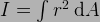where$r$ is the distance of the area element$\mathrm dA$ from the axis of rotation. The unit of polar moment of inertia is m4.

Polar second moment of area is often confused with the area second moment of inertia, which is defined: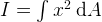where$x$ is the distance of the area element$\mathrm dA$ from a specific plane.  This also has units of m4, however physically this quantity indicates the resistance of an object to bend about a certain plane when subjected to a torque.

## Mathematical definition of Moment of Inertia and Polar Moment of Inertia:

Moment of inertia is defined as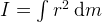Polar moment of inertia is defined as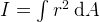## Physical Meaning:

Moment of inertia is a measurement of an object’s resistance to angular acceleration.

Polar moment of inertia is a measurement of an object’s resistance to torsion (twisting).

## Units:

Moment of inertia is measured in units of kg m2.

Polar moment of inertia is measured in units of m4.

### Feedback is important to us.

error: Content is protected !!